## Matching Supply with DemandLooking for Management Homework Help?Sustainability is one of the most important aspects of the modern businesses. Sustainability, on one hand, works on efficient uses of the resources and on the other side, emphasizes on reduction of total emission per unit of output produced. The fuel oil emits 38.2 kg of CO2 per litre of fuel and the fuel used for transportation is 1,400,000 litres. So,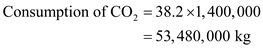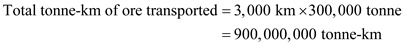Compute the emission as follows:So, the emission of CO2 is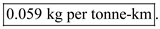The breakeven point between two alternatives happens at a time point when the total cost (i.e. the sum of all fixed and variable cost) becomes the same for both the alternatives. a. Let us define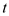as the time in hours when the breakeven occurs for the LED. Compute the total cost of using one LED for t hours as follows: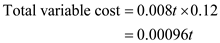Purchase cost of one LED = \$12 So,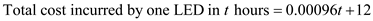--- (1) Compute the total cost of using one light bulb for t hours as follows: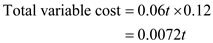Purchase cost of one bulb = \$0.4 So,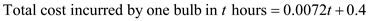---- (2) For breakeven to happen, equate equation (1) and (2) as follows:However, note that this value is more than 1,000 hours which is the life of one bulb. So, it is not possible to take this value as the breakeven hours. Re-compute the breakeven by considering two bulbs purchased i.e. double the purchase cost from \$0.40 to \$0.80.Rounding off, the total hours after which the LED will become breakeven with the bulb is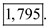b. First, compute the total hours of operation in one year.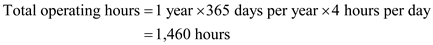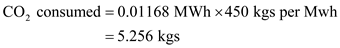So, in a year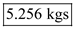of CO2 will be consumed by LED.